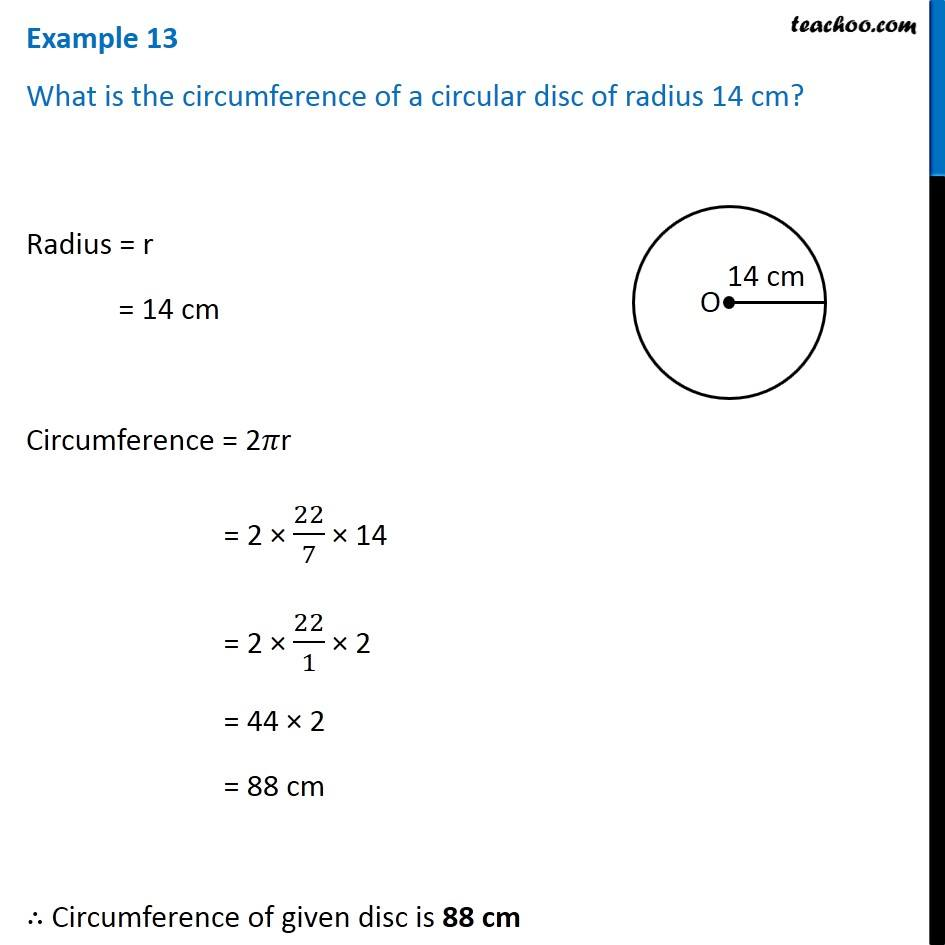1. Chapter 11 Class 7 Perimeter and Area
2. Concept wise
3. Circumference of circle

Transcript

Example 13 What is the circumference of a circular disc of radius 14 cm? Radius = r = 14 cm Circumference = 2𝜋r = 2 × 22/7 × 14 = 2 × 22/1 × 2 = 44 × 2 = 88 cm ∴ Circumference of given disc is 88 cm